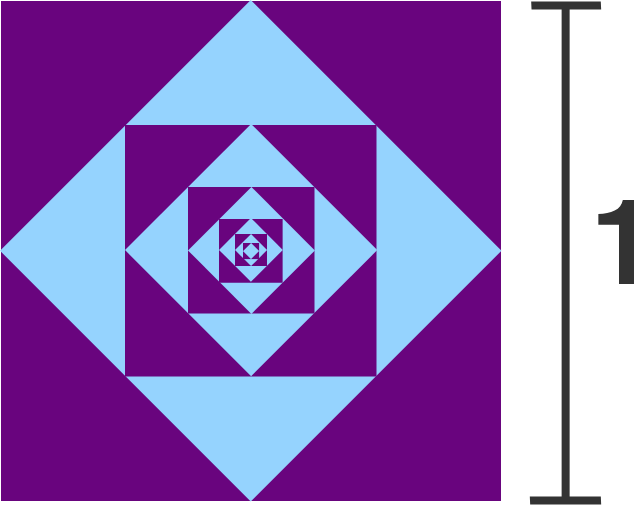# 58 of 100: Squares Within Squares Within Squares...

Geometry Level 1Starting with a unit square, a series of smaller squares is made by repeatedly connecting the midpoints of the sides of each square in turn. Given how the pattern is shaded, approximately what total area is shaded purple?An approximation is good enough to solve this puzzle; more advanced solvers should do a proof! And you don't need to add up infinitely many fractions to find the answer, there's a faster way!

×